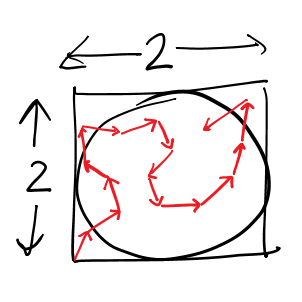# A Drunkard can Help Estimate the Value of Pi

Monte Carlo method with direct sampling helps us reason about probabilistic systems. The method is straightforward, we generate a sample input based on the respective probability distribution and analyze the output appropriately. However, there are situations when direct sampling is not feasible and the method cannot be applied off-the-shelf. For example, a case when the probability distribution of the inputs is not available. But, we are given certain rules to iterate over the sample space, e.g., a random walk, and we need to estimate the desired properties using the same.

Consider an example of a drunkard who keeps walking randomly. The drunkard at any position takes a step in a random direction of fixed length and keeps repeating the same. This is in contrast with the example of my other post on Monte Carlo methods. Note that we are not given the distribution of the points he visits, however, we are given the rules to compute the probability of his next step. These random walks are formally called Markov chains. A Markov chain describes a sequence of stochastic events where the probability of the next event is only dependent on the current state.

Let us conduct an experiment with the drunkard’s random walk. We take a 2x2 square bounding a circle of radius 1 centred at (0, 0). We let the drunkard move in the square starting from (-1, -1), and at each step, we count the number of times the drunkard lies in the circle (points_in). For a fairly large number of steps (N), the value (4*points_in/N) starts converging to pi.Following python program simulates the experiment. At each step, the new point (x_new, y_new) is computed relative to the last position by adding a random vector to it. If the new point lies outside the square, then we do not take the step. We count the number of times the drunkard lies in the circle with the variable points_in. Finally, the program prints the value (4*points_in/N) after N steps.

import random, math

x, y = -1, -1
delta = 0.1 # length of step taken
points_in = 0
N = 1000000
for step in range(N):
theta = random.uniform(0, 360) # generate a random angle
x_new = x + delta * math.cos(theta)
y_new = y + delta * math.sin(theta)
# do not move if new point is out of square
if -1 < x_new < 1 and -1 < y_new < 1:
(x, y)  = (x_new, y_new)
if x**2 + y**2 < 1: # in the circle
points_in += 1
print(4*points_in/N)


Following are the three values that I got by the running the program three times: 3.121184, 3.141716, 3.137896. Note that values are close to the value of pi. Increasing the value of N results in the values closer to the value of pi.

The reader must be curious, why does this scheme work. What kind of sampling does the random walk generate? Why does increasing the value of N result in a better estimate of pi? Why does the value converge to pi? I plan to note down all the ideas involved, and finally, answer all the questions raised. Stick to this space for the detailed answers!